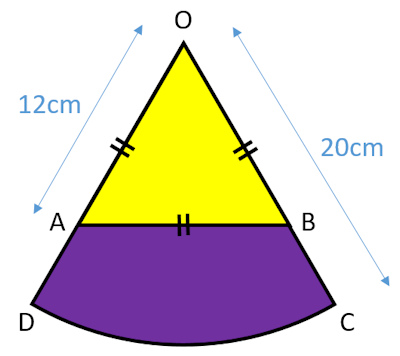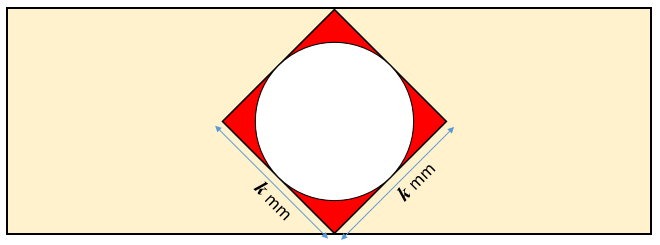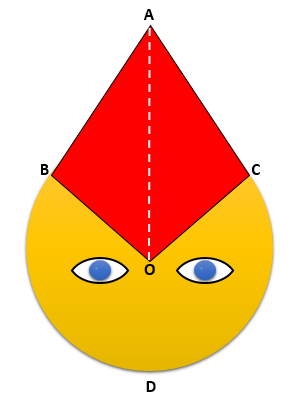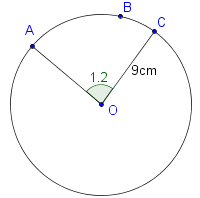# Exam-Style Questions.

## Problems adapted from questions set for previous Mathematics exams.

### 1.

GCSE HigherThe diagram shows a sector of a circle, centre O, with one angle shown as being 110°. If the radius of the circle is 8cm calculate:

(a) The area of the shaded area.

(a) The perimeter of the shaded area.

### 2.

GCSE Higher

A yellow equilateral triangle has been painted on a purple sector. The side OC is 20cm and OA is 12cm. Calculate the area of the purple region ABCD as a percentage of the area of the whole sector OCD.The diagram is not drawn to scale.

### 3.

IGCSE ExtendedA circle is drawn inside a square so that it touches all four sides of the square.

(a) If the sides of the square are each $$k$$ mm in length and the area of the red shaded region is $$A$$ mm2 show that:

$$4A=4k^2-\pi k^2$$

(b) Make $$k$$ the subject of the formula $$4A=4k^2-\pi k^2$$

### 4.

GCSE HigherThis draft emoji design (not drawn to scale) includes a circle and a kite.

AB and AC are both tangents to the circle centre O.

The radius of the circle is 7cm. The dashed line AO is 12cm.

Calculate the length of the arc BDC.

### 5.

GCSE Higher

Three crayons are held together with an elastic band. The diagram below shows the end of the crayons and the elastic band.Each of the crayons has diameter of 10 mm. Find the length of the elastic band in this position

### 6.

IB Standard

The following diagram shows a circle with centre O and radius 9 cm.The points A, B and C lie on the circumference of the circle, and AÔC = 1.2 radians.

(a) Find the length of the arc ABC.

(b) Find the perimeter of the minor sector OAC.

(c) Find the area of the minor sector OAC.

### 7.

GCSE Higher

Two identical small yellow circles are drawn inside one large circle, as shown in the diagram. The centres of the small circles lie on the diameter of the large circle. The part of the large circle that is outside both small circles is painted red.Find the fraction of the large circle that is painted red.

The exam-style questions appearing on this site are based on those set in previous examinations (or sample assessment papers for future examinations) by the major examination boards. The wording, diagrams and figures used in these questions have been changed from the originals so that students can have fresh, relevant problem solving practice even if they have previously worked through the related exam paper.

The solutions to the questions on this website are only available to those who have a Transum Subscription.

Exam-Style Questions Main Page

Search for exam-style questions containing a particular word or phrase:

To search the entire Transum website use the search box in the grey area below.